Categories

# Compressed storage matrix

Foreword

A program into the deep sea, from bricks lover, moving day, moving every night, moved forever, exhausted and death of people, straight people lose themselves, forget the time, suddenly found that between September running out, quickly write a blog to make a card to prove it I’m still alive. . .

Array and Matrix

An array is a sequence composed of a limited set of data elements of the same type, access to data elements of each sequence number is used to access the elements, i.e. the subscript. Array itself is a generalization of linear form, is a one-dimensional array in the form of a linear vector table, linear table consists of two-dimensional array is an array of one-dimensional.

In many scientific and engineering computing applications, often use the concept of matrix, in fact, most of Mysql tables, table data is stored in the ranks with us, which is the matrix.

Since the matrix having a fixed number of elements and superscript elements pressed ordered relationship, etc., so when using high-level programming languages, it is generally used to store a two-dimensional matrix array.

Sequential storage array

Why are stored sequentially?

I want to ask this question too low level. Because it is an array of data storage into the chain stores two sequential storage and, once the array is defined, his community had dimensions and fixed dimensions, in addition to initialization and destruction of the structure, the array will have access operating elements and modifying elements, insertion and deletion operations does not exist, so the array for storing sequence.

Array stored in memory mapping relations

Arrays can be multi-dimensional, but the memory space is one-dimensional, so we take a multidimensional array through certain mapping order to turn it into a one-dimensional, then stored into the memory space.

In most high-level programming languages, multidimensional arrays in memory are usually two different storage order, rows and columns priority storage priority storage.

For example, the line 3 a two-dimensional array of matrix 4:

a1,a2,a3,a4
b1,b2,b3,b4
c1,c2,c3,c4

Row-priority storage: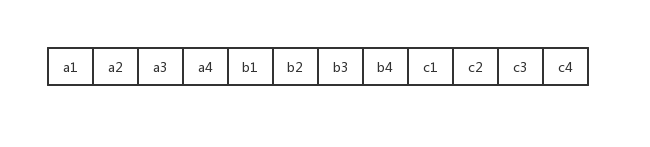Priority storage columns: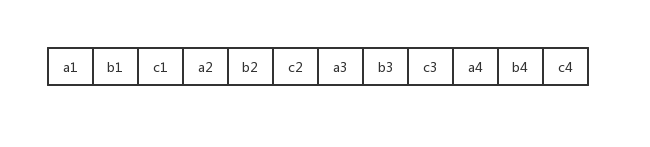Address calculation means that for a given array subscript, seeking in a one-dimensional address space of the memory, the data thus extracted.

We address first look one-dimensional array of computing

Elements within only a one-dimensional array index, and storage methods as ordinary linear form.

As one-dimensional array A = [a1, a2, a3, …… ai, ………, an], each element occupies size memory cells (that is, memory size), then the elements ai memory address is a  position + (i-1) * size

Let’s look at two-dimensional array address calculation

Amn two-dimensional array as an example, is the first element A  , any element of the array A [i] [j] of the address is: A   position + (n * (i- 1) + (j-1)) * size;

For example: a two-dimensional array A 4 line 5 columns, row-wise, wherein each memory cell occupies two elements, the first element address is 1000, seeking row 3, column 2 in the address memory elements.

We parameters set into the formula, the answer = 1000 + (4 * (3-1) + (2-1)) = 2 * 1018;

If the matrix on paper observed a glance: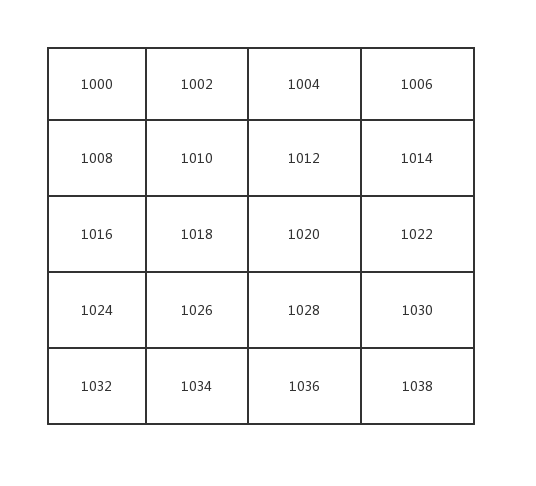Content formula is the number of lattice obtained by multiplying each grid storage unit occupied, coupled’s first address.

Matrix transpose

Design an algorithm of a matrix A (m * n) is the transposed matrix of B (n * m), simply put, is the interchanging rows.

```\$arr = [
['张三','男','北京'],
['李四','女','上海'],
['王五','男','广州'],
];

function transpose(\$a){
\$b = [];
for (\$i = 0;\$i < count(\$a); \$i ++){
for(\$j = 0;\$j < count(\$a[\$i]); \$j ++){
\$b[\$j][\$i] = \$a[\$i][\$j];
}
}
return \$b;
}

\$result = transpose(\$arr);```

Results: \$ result = [[ ‘John Doe’, ‘John Doe’, ‘king of five’], [ ‘male’, ‘female’, ‘M’], [ ‘Beijing’, ‘Shanghai’, ‘Guangzhou’ ],];

Special Matrices

Special compressed storage matrix

It refers to the special matrix having many of the same element or elements to zero, and the distribution of these elements in the matrix have a certain regularity.

If such a matrix is ​​also used to store the preceding embodiment, it will generate a lot of wasted space, in order to save storage space and to be of such a matrix a compressed storage, compression and storage method is to render the elements the same as those allocated only regular distribution a storage space for zero elements does not allocate storage space.

Triangular matrix

We do the following triangular matrix triangle example, as shown: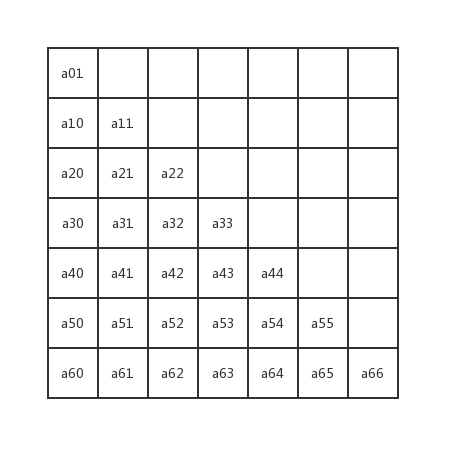Of all the spaces are filled with data or null constants are the same, that is, the spaces are all the same data.

The case where row stored, the size of the one-dimensional memory storage space is: 1 + 2 + 3 + 4 + 5 + 6 + 7 = n (n + 1) / 2 = 7 * (7 + 1) / 2 = 28, of course, finally, adding a storage space for storing data on the same triangle.

Then for any element of aij, one-dimensional address space in the storage memory is still calculated lattice obtained depends, to calculate the total number of rows of the grid occupy, plus the number of rows of the grid current: a   position + (i * (i + 1) / 2 + j) * size;

We use a formula to verify, a42 where the number of grid = (i * (i + 1) / 2 + j) = 4 * 5/2 + 2 = 12

Band matrix

Band matrix also called a diagonal matrix, as shown: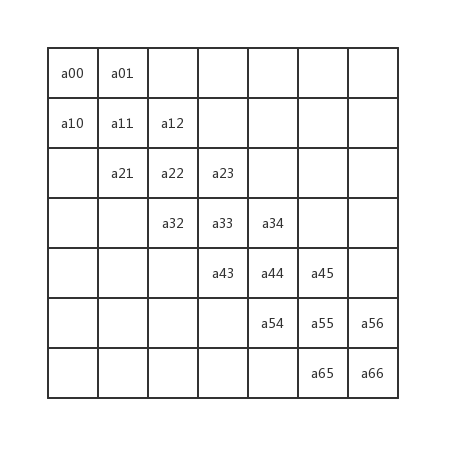Characterized in that the band matrix: all non-zero elements are concentrated on three diagonals region centered on the main diagonal elements are 0 in other regions.

In addition to the first and last rows only two non-zero elements, the remaining rows are three non-zero elements, in other words, each row is three non-zero elements, but less a first row, the last row less the one, the one-dimensional space is required: 3n – 2;

Then for any element aij, how to calculate it in memory address space it?

After that i and j can be observed in the vicinity of the diagonal, with the distribution of the results subtracted are as follows

j – i = 1; diagonal upper j – i = 0; diagonal j – i = -1; below the diagonal

No matter which position in the diagonal, we can all use a common approach to calculate the address, which is the first to calculate the percentage of the grid above the line, coupled with the grid of the current line.

The number of rows above: i, since the beginning of row 0 are counted, the number of lines i is above this value.

The above grid number: 3 * i – 1, 1 is reduced because at least a first grid line.

Current row grid number: j – i + 1; i and j, the relationship, a value plus 1 after subtraction we obtain lattice current row number.

Then the last memory address aij = a00 first address + ((3 * i -1) + (j-i + 1)) * size; size is the size of each memory cell occupied by the data.

For example the first address of 1000, data of each memory cell occupies two, then the address in memory a45 = 1000 + 13 * 2 = 1026;

Sparse matrix of compressed storage

Because of the special distribution of non-zero elements of the matrix is ​​regular, so you can always find under the matrix elements with the subject of correspondence between the one-dimensional array, but there is a matrix, the matrix most elements are zero, non-zero in general only 30% of the total number of elements of the matrix elements, and the elements are distributed without any rules, so we called a sparse matrix.

If the conventional method of storing sparse matrix, will be quite a waste of storage space, so we need to store only nonzero elements. Since the non-zero elements in the sparse matrix distribution is not regular, so in addition to the value stored in the non-zero elements, we need to store non-zero elements in row, column position, i.e. the triple (i, j, aij ).

Figure:The so-called triplets, i.e. a matrix, a two-dimensional array, each row three columns, respectively, row number, column number, element values.

After due triples between the sparse matrix memory address plays the role of a middleman, so sparse matrix of compressed storage, it lost the characteristics of random access.